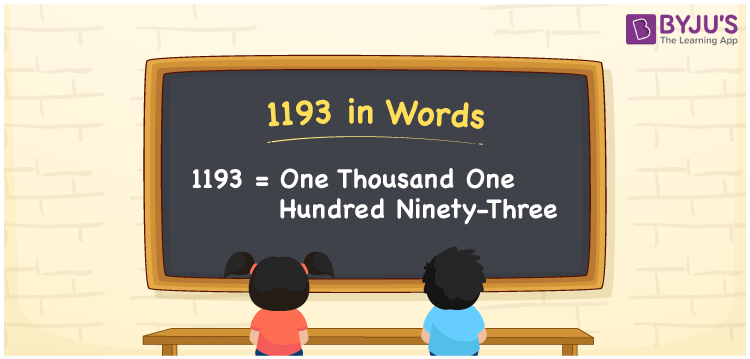# 1193 in Words

We can write 1193 in words as One thousand one hundred ninety-three. Suppose you have noted Rs. 1193 as an expense for medicines, then you may read it as “The expense on medicines is One thousand one hundred ninety-three rupees”. In this article, you will learn how to convert the cardinal number 1193 into words in an easily understandable method.

 1193 in words One thousand one hundred ninety-three One thousand one hundred ninety-three in Numbers 1193

## 1193 in English Words

Generally, we express numbers in words using the English alphabet. So, we can spell 1193 in English as “One thousand one hundred ninety-three”.## How to Write 1193 in Words?

The below table shows the place values for all the four digits of the number 1193. Using these values, we can convert the number 1193 to words. This can be done as explained below.

 Thousands Hundreds Tens Ones 1 1 9 3

Here, ones = 3, tens = 9, hundreds = 1, and thousands = 1.

Let us expand these numbers with respect to the place values.

1 × Thousand + 1 × Hundred + 9 × Ten + 3 × One

= 1 × 1000 + 1 × 100 + 9 × 10 + 3 × 1

= 1000 + 100 + 90 + 3

= One thousand + One hundred + Ninety + Three

= One thousand one hundred ninety-three

Thus, 1193 in words = One thousand one hundred ninety-three.

1193 is a natural number that precedes 1194 and succeeds 1192.

1193 in words – One thousand one hundred ninety-three

Is 1193 an even number? – No

Is 1193 an odd number? – Yes

Is 1193 a composite number? – No

Is 1193 a prime number? – Yes

Is 1193 a perfect square number? – No

Is 1193 a perfect cube number? – No

## Frequently Asked Questions on 1193 in Words

Q1

### How do you spell 1193?

The spelling of 1193 in English is One thousand one hundred ninety-three. Hence, we can spell 1193 as One thousand one hundred ninety-three.
Q2

### How to write Rs. 1193 in words on a cheque?

On a cheque, we generally write an amount of Rs. 1193 in words as “One thousand one hundred ninety-three rupees only”.
Q3

### Express the value of 1 + 1193 in words.

1 + 1193 = 1194 = One thousand one hundred ninety-four.